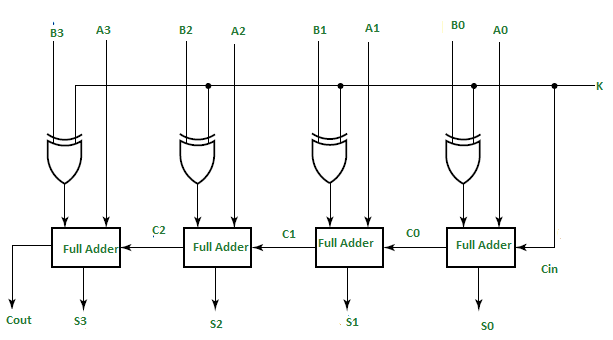In Digital Circuits, A Binary Adder-Subtractor is one which is capable of both addition and subtraction of binary numbers in one circuit itself. The operation being performed depends upon the binary value the control signal holds. It is one of the components of the ALU (Arithmetic Logic Unit).

This Circuit Requires prerequisite knowledge of Exor Gate, Binary Addition and Subtraction, Full Adder.

Lets consider two 4-bit binary numbers A and B as inputs to the Digital Circuit for the operation with digits

```A0 A1 A2 A3 for A
B0 B1 B2 B3 for B ```

The circuit consists of 4 full adders since we are performing operation on 4-bit numbers. There is a control line K that holds a binary value of either 0 or 1 which determines that the operation being carried out is addition or subtraction.As shown in the figure, the first full adder has control line directly as its input(input carry C0), The input A0 (The least significant bit of A) is directly input in the full adder. The third input is the exor of B0 and K (S in fig But do not confuse it with Sum-S). The two outputs produced are Sum/Difference (S0) and Carry (C1).

If the value of K (Control line) is 1, th output of B0(exor)K=B0′(Complement B0). Thus the operation would be A+(B0′). Now 2’s complement subtraction for two numbers A and B is given by A+B’. This suggests that when K=1, the operation being performed on the four bit numbers is subtraction.

Similarly If the Value of K=0, B0 (exor) K=B0. The operation is A+B which is simple binary addition. This suggests that When K=0, the operation being performed on the four bit numbers is addition.

Then C0 is serially passed to the second full adder as one of it’s outputs.The sum/difference S0 is recorded as the least significant bit of the sum/difference. A1, A2, A3 are direct inputs to the second, third and fourth full adders.Then the third input is the B1, B2, B3 EXORed with K to the second, third and fourth full adder respectively. The carry C1, C2 are serially passed to the successive full adder as one of the inputs. C3 becomes the total carry to the sum/difference. S1, S2, S3 are recorded to form the result with S0.

For an n-bit binary adder-subtractor, we use n number of full adders.

Example:
Lets take two 3 bit numbers A=010 and B=011 and input them in the full adder with both values of control lines.

```For K=0:
B0(exor)K=B0 and C0=K=0

= A0+B0
= 0+1
= 1,

S0=1
C1=0
Similarly,
S1=0 with C2=1
S2=1 and C2=0

Thus,
A = 010 =2
B = 011 = 3
Sum = 0101 = 5

For K=1
B0(exor)K=B0' and C0=k=1

Thus
S0=1 and C1=0
Similarly
S1=1 and C2=0
S3=1 and c3=1

Thus,
A = 010 = 2
B = 011 = 3
Sum(Difference) = 1111 = -1 ```

Attention reader! Don’t stop learning now. Get hold of all the important CS Theory concepts for SDE interviews with the CS Theory Course at a student-friendly price and become industry ready.

My Personal Notes arrow_drop_upCheck out this Author's contributed articles.

If you like GeeksforGeeks and would like to contribute, you can also write an article using contribute.geeksforgeeks.org or mail your article to contribute@geeksforgeeks.org. See your article appearing on the GeeksforGeeks main page and help other Geeks.

Please Improve this article if you find anything incorrect by clicking on the "Improve Article" button below.